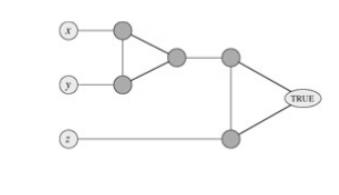Home | | User Interface Design | Graph Coloring

# Graph Coloring

A coloring of a graph is an assignment of a color to each vertex of the graph so that no two vertices connected by an edge have the same color. It is not hard to see that our problem is one of coloring the graph of incompatible turns using as few colors as possible.

GRAPH COLORING

A coloring of a graph is an assignment of a color to each vertex of the graph so that no two vertices connected by an edge have the same color. It is not hard to see that our problem is one of coloring the graph of incompatible turns using as few colors as possible.

The problem of coloring graphs has been studied for many decades, and the theory of algorithms tells us a lot about this problem. Unfortunately, coloring an arbitrary graph with as few colors as possible is one of a large class of problems called "NP-complete problems," for which all known solutions are essentially of the type "try all possibilities."

A k-coloring of an undirected graph G = (V, E) is a function c : V → {1, 2,..., k} such that c(u) ≠ c(v) for every edge (u, v) E. In other words, the numbers 1, 2,..., k represent the k colors, and adjacent vertices must have different colors. The graph-coloring problem is to determine the minimum number of colors needed to color a given graph.

a.                 Give an efficient algorithm to determine a 2-coloring of a graph if one exists.

b.                 Cast the graph-coloring problem as a decision problem. Show that your decision problem is solvable in polynomial time if and only if the graph-coloring problem is solvable in polynomial time.

c.                  Let the language 3-COLOR be the set of graphs that can be 3-colored. Show that if 3-COLOR is NP-complete, then your decision problem from part (b) is NP-complete.

To prove that 3-COLOR is NP-complete, we use a reduction from 3-CNF-SAT. Given a formula φ of m clauses on n variables x, x,..., x, we construct a graph G = (V, E) as follows.

The set V consists of a vertex for each variable, a vertex for the negation of each variable, 5 vertices for each clause, and 3 special vertices: TRUE, FALSE, and RED. The edges of the graph are of two types: "literal" edges that are independent of the clauses and "clause" edges that depend on the clauses. The literal edges form a triangle on the special vertices and also form a triangle on x, ¬x, and RED for i = 1, 2,..., n.

d.                 Argue that in any 3-coloring c of a graph containing the literal edges, exactly one of a variable and its negation is colored c(TRUE) and the other is colored c(FALSE).

Argue that for any truth assignment for φ, there is a 3-coloring of the graph containing just the literal edges.

The widget is used to enforce the condition corresponding to a clause (x y z). Each clause requires a unique copy of the 5 vertices that are heavily shaded; they connect as shown to the literals of the clause and the special vertex TRUE.

e.                  Argue that if each of x, y, and z is colored c(TRUE) or c(FALSE), then the widget is 3-colorable if and only if at least one of x, y, or z is colored c(TRUE).

f.                   Complete the proof that 3-COLOR is NP-complete.Fig: The widget corresponding to a clause (x  y z), used in Problem

Study Material, Lecturing Notes, Assignment, Reference, Wiki description explanation, brief detail
Analysis and Design of Algorithm : Backtracking : Graph Coloring |